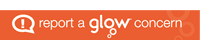UoDEdushare

The central sharing hub for #UoDedu teacher education students at the University of DundeeNovember 25, 2017
by Rachel Noble
Comments Off on Reflection post on science and maths!

Reflection post on science and maths!

What links does maths have with science? In class, we drew graphs to plot y=x, y=x^2, y=1/x and y=1/x^2. In order to draw these graphs, we first needed a fundamental understanding of basic concepts such as numbers, the sequence of numbers and being able to understand patterns in order to compare results and see […] Continue reading